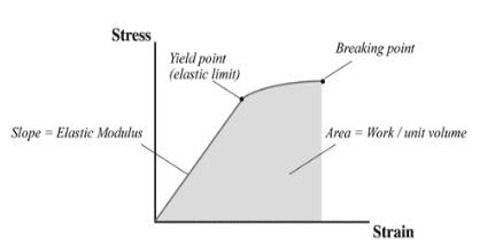Physics

# Moduli of ElasticityModuli of Elasticity

We get from Hooke’s law that within elastic limit the ratio of stress and strain is a constant. This constant is called coefficient of elasticity or elastic constant. Moduli of Elasticity mean any of several coefficients of elasticity of a body, expressing the ratio between a stress or force per unit area that acts to deform the body and the corresponding fractional deformation caused by the stress. It the ratio of the stress applied to a body or substance to the resulting strain within the elastic limit. The modulus of elasticity of a material is a measure of its stiffness and for most materials remains constant over a range of stress.

Definition: Within elastic limit the ratio of stress and strain is a constant. This constant is called coefficient of elasticity of the material of the body.

So, coefficient of elasticity, E = stress / strain

Both stress and strain are scalar quantities, so coefficient of elasticity is also scalar quantity.

Dimension: Dimension of elastic constant, [E] = ML-1T-2

Unit: Unit of coefficient of elasticity is Nm-2 or Pa

From the discussion of stress and strain we find that due to the variation of stress and strain elastic coefficients are of three types, viz- (1) Young’s modulus, (2) Rigidity modulus and (3) Bulk or volume modulus.